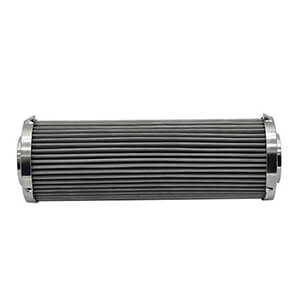# Filter pressure drop design

Aug. 08, 2018Filter pressure drop is what we should know when we use the product. What is it?

The term “Pressure Drop” gets used a lot in the indoor air quality industry, but it isn’t always well defined. The Wikipedia definition of pressure drop is as follows:

Pressure drop is a term used to describe the decrease in pressure from one point in a pipe or tube to another point downstream. When air is drawn through a system with a filter installed, that filter will create some resistance to the airflow. As a result, the difference in air pressure in the ductwork on one side of the filter versus the other side of the filter is what professionals refer to as pressure drop.

The filter element should be designed to have a large flow rate and a small pressure drop.

1. Filter pressure drop of woven mesh filter

The filter pressure drop of the woven wire mesh filter is calculated according to the formula①

△P1=1/2ε(Q/A0)2ρ         ①

In formula

△P1-Filter pressure drop, Pa;

Q-rate of flow, m3/s;

P- Fluid density, kg/m3;

A0-Filter through hole area, m2;

ε- resistance coefficient.

When Reynolds number is Re=Vdw/V≧400, resistance coefficient Calculated according to formula  ②

ζ=1.3(1-A0/A)+(A/A0-1)2  ②

In formula

A-Filtration area, m2;

A v-flow rate of liquid through the filter element, m/s;

DW-Mesh diameter, m.

When Reynolds is﹤400,εTaking the value εˊ and calculating according to the formula ③

εˊ=ηε

In formula

η-The correction factor affected by Re is shown in table ④

Table④   The correction factor affected by Re

 Re 50 100 150 200 300 400 η 1.44 1.24 1.13 1.08 1.03 1.01

For multi-layer mesh filters, the resistance coefficient should be taken Σε1, when calculating the pressure drop resistance.(ε1 is the multi-layer resistance coefficient)

2. Filter pressure drop of stainless steel fiber felt

Filter element pressure drop made of stainless steel fiber felt which calculated according to the formula⑤

△P1=27.3×Qμ/A×H/K

In formula

μ-Hydrodynamic viscosity, Pa·S;

H-Filter fiber felt thickness, m;

K-Permeability coefficient, m³,(See table⑥)

Table ⑥    The permeability coefficient of filter fiber made by Belgium

 The filter material grade ST3AL3 T5AL3 T7AL3 ST10AL3 T15AL3 Permeability coefficient K 0.53×10﹣12 1.61×10﹣12 2.67×10﹣12 5.78×10﹣12 11.0×10﹣12

3. Filter pressure drop of metal powder sintered filter element

The pressure drop of the metal powder sintered filter element is calculated according to the formula⑦

△P1=Qμ/KA×106   ⑦

In formula

K1-Filtration capacity coefficient.

Filtration capacity coefficient is calculated according to the formula⑧

Kˊ=1.04d22×10³/ts ⑧

In formula

the d2-Average diameter of sintered powder particles, m;

ts-Sintered plate thickness,m.

4. Filter pressure drop of metal wire wound filter

The pressure drop of metal wire wound filter is calculated according to the formula⑨

△P1=Qμ/πdsL1×Kx×106     ⑨

In formula

a ds-The diameter of the outer circle of the winding frame, m;

L1-Winding core length,m;

Kx=The filtration capacity coefficient of the winding filter element(Kx=5.88m﹣1).

5. Pressure drop of fiber filter element

The pressure drop of the fiber filter element is calculated according to the formula⑩

△P1=Qμ/A×Kx×108         ⑩

Total filtration capacity of the filter element made of fiber filter material Kx=1.67﹣1m, Including plant fiber, fiberglass, and non-woven fabric.

Air filtration consumes energy in the way that conditioning coils do; however, the filters' energy consumption is greater because their resistance to air flow increases over time by definition. Specifying air filters with the lowest possible pressure drop nearly always pays for itself. Generally, the pressure drop is a direct function of the filtration efficiency; in other words, high-efficiency filtration results in a high-pressure drop.